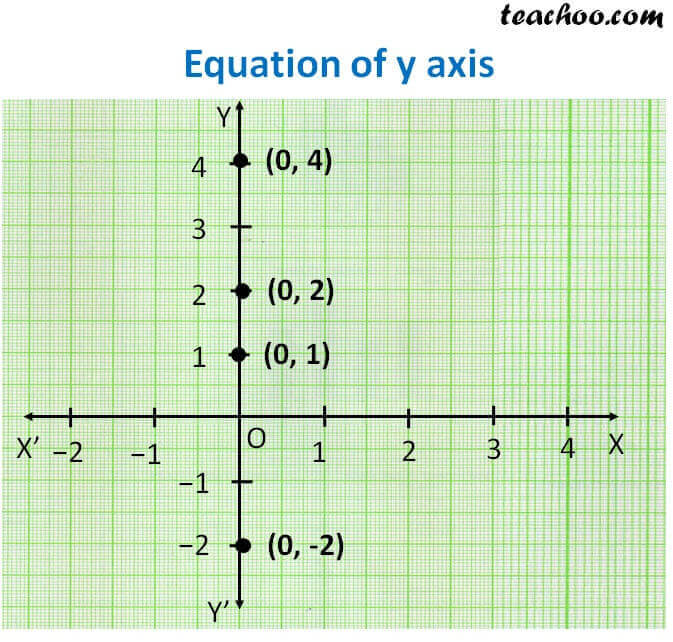Lines parallel x or y axis

Chapter 4 Class 9 Linear Equations in Two Variables
Concept wise

Let's plot  (0, 1) (0, 2) (0, 4) (0, −2) on the graphAll points in y-axis are like

(0, 1) (0, 2) (0, 4) (0, −2)

So x = 0 for all points in y-axis

∴ Equation of y axis is x = 0

Learn in your speed, with individual attention - Teachoo Maths 1-on-1 Class